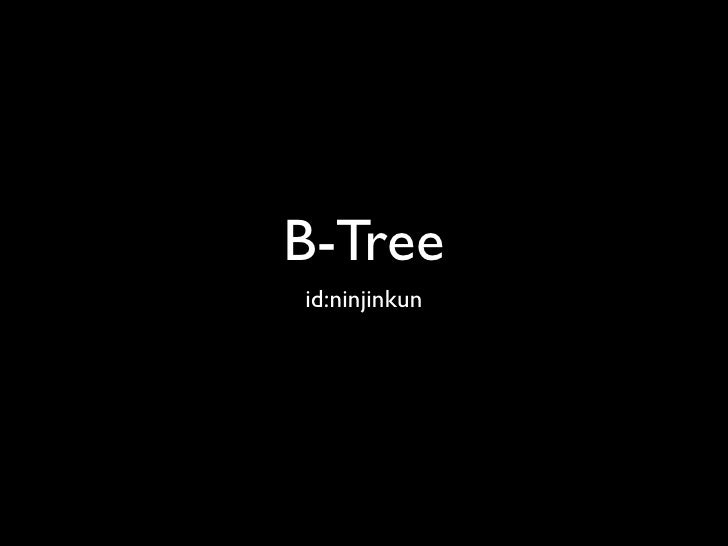Successfully reported this slideshow.Upcoming SlideShare
×

# Algorithm Introduction #18 B-Tree

3,653 views

Published on

はてなで行われたアルゴリズム・イントロダクション勉強会第18章B-Treeの資料です．

Published in: Technology
• Full Name
Comment goes here.

Are you sure you want to Yes No### Algorithm Introduction #18 B-Tree

1. 1. B-Tree id:ninjinkun
2. 2. B- • Multiway Balanced Tree ( ) Key Feature • • Application • DB 2
3. 3. B- 3
4. 4. • HDD 5,400-15,000[RPM] • 7300RPM 1 8.33[ms] • RAM 0.00001 [ms] HDD 3~9 [ms] SSD 0.1 [ms] 4
5. 5. • • (211~214 ) • • • CPU • 5
6. 6. B- • • • 6
7. 7. B- 7
8. 8. B- 1. x n[x] a. x n[x] b. c. x TRUE FALSE leaf[x] 8
9. 9. B- 2. x n[x] + 1 ci 9
10. 10. B- 3. N[x] key[x] c[x] k 10
11. 11. B- 4. h 11
12. 12. B- 5. 1 B- t >2 a. t-1 t b. 2t - 1 1 2t 12
13. 13. • • n >1 t >2 B- h T 13
14. 14. • 1 t-1 2th-1 logt 14
15. 15. B- • 2 • O(lg n) • 2 lg t 15
16. 16. B- • B-TREE-SEARCH, B-TREE-CREATE, B-TREE- INSERT • • DISK-READ DISK-WRITE • DISK- READ 16
17. 17. B- 3 5 9 12 17
18. 18. B- 3 5 9 12 17
19. 19. B- 3 5 9 12 17
20. 20. B- 3 5 9 12 10 11 17
21. 21. B- 3 5 9 12 10 11 17
22. 22. B- • B- h, n O(h) = O(logtn) • n[x] < 2t O(t) • CPU O(th) = O(tlogtn) 18
23. 23. B- 19
24. 24. B- • • • • y • http://slady.net/java/bt/view.php?w=800&h=600 20
25. 25. B- …NW… …NW… …NW… 21
26. 26. B- …NW… 22
27. 27. B- 23
28. 28. B- x X 24
29. 29. B- 25
30. 30. B- 1. x 2. x a. yt 26
31. 31. B- a. y k a. b. k z t b. z k 27
32. 32. B- b. c. 3. a. b. 28
33. 33. B+-Tree • • • 29
34. 34. B-Tree • B#-Tree • Search • Insert • B*-Tree • , 3 30